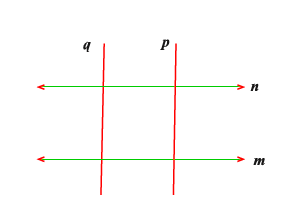# Prove that two lines are respectively perpendicular to two parallel lines , they are parallel to each other.Given:

Two lines m and n are parallel and another two lines p and q are respectively perpendicular to m and n.

Or 𝑝⊥𝑚 and 𝑝⊥𝑛,𝑞⊥𝑚 and 𝑞⊥𝑛

To prove: 𝑝∥𝑞

Proof:

Since, 𝑚∥𝑛 and p is perpendicular to m and n.

So,

p is perpendicular to m …(i)

p is perpendicular to n …(ii)

Since, 𝑚∥𝑛 and q is perpendicular to m and n.

So,

q is perpendicular to m …(iii)

q is perpendicular to n …(iv)

From the equations (i) and (iii) [or from (ii) and (iv)], we get 𝑝 ∥𝑞. [If two lines are perpendicular to the same line, lines are parallel to each other] Hence, 𝑝 ∥𝑞.

Updated on: 10-Oct-2022

74 Views